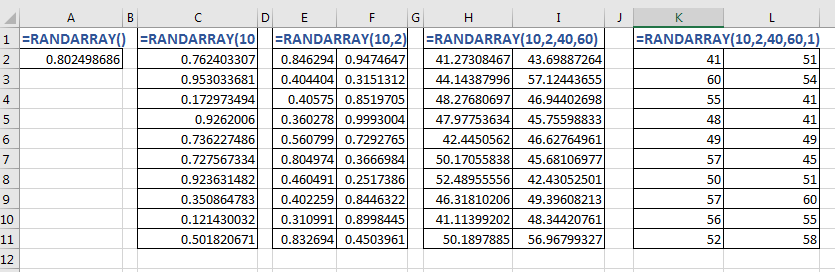# Excel RANDARRAY Function

In Excel 2019 and 365 we get a new function called RANDARRAY. This is a dynamic array function that returns an array of random numbers. In Excel 2016 and older versions, we have the RAND and RANDBETWEEN functions. But this function can do work for both of these functions. How? Let's first have a look at the syntax of the RANDARRAY function.Syntax of the RANDARRAY function

=RANDARRAY([rows],[columns],[min],[max],[integer])

All the arguments in this function are optional. If you don't give any arguments to the function, it will work as the RAND function and will return one fractional random number between 1 and 0.

[rows]: The number of numbers that you want vertically (number of rows you want fill).

[columns]: The number of numbers that you want horizontally (number of columns you want fill).

[min]: The starting number or the minimum value of the random number/s.

[max]: The maximum range of the number.

[integer]: Set it true, if you want the random numbers to be whole numbers. By default, it is false and returns fractional random numbers/

Using RANDARRAY Function
So if you just want a random fractional number between 1 and 0, write this formula:

=RANDARRAY()

If you want an array of 10 fractional numbers spilled downwards, write this formula:

=RANDARRAY(10)

As soon as you hit the enter button, 10 rows below will be filled with 10 random fraction numbers, since it is a dynamic array function.
If you want an array of 20 fractional numbers spilled in 10 rows downwards and 2 columns to the right, write this formula:

=RANDARRAY(10,2)

This time array is spilled into two columns and 10 rows.

If you want an array of 20 fractional numbers between 40 and 60, spilled in 10 rows downwards and 2 columns to the right, write this formula:

=RANDARRAY(10,2,40,60)

The array returned by this function restricts to the range 40 and 60.

If you want an array of 20 whole numbers spilled in 10 rows downwards and 2 columns to the right, write this formula:

=RANDARRAY(10,2,40,60,1)

This time we tell the RANDARRAY function to return only integer random numbers in the array.

So yeah guys, this is how we use the RANDARRAY function in Excel. This function is new and not available in Excel 2016 and older versions.

I hope it was explanatory enough. If you have any doubts regarding this article or any other Excel VBA topic, ask in the comments section below.

Related Articles:

Excel Random Selection: How to Get Random Sample From a Dataset | To select random data from a data set, we use the RANDBETWEEN function and the INDEX function.

Excel RANDBETWEEN Function | The Excel RANDBETWEEN function returns a random number between specified range.

Get Random number From Fixed Options |  To get random numbers from a fixed range we use this Excel Formula.

How to Generate Random text values in Excel | To Generate random text in Excel, we use CHOOSE and RANDBETWEEN function.

Popular Articles:

50 Excel Shortcuts to Increase Your Productivity | Get faster at your task. These 50 shortcuts will make you work even faster on Excel.

The VLOOKUP Function in Excel | This is one of the most used and popular functions of excel that is used to lookup value from different ranges and sheets.

COUNTIF in Excel 2016 | Count values with conditions using this amazing function. You don't need to filter your data to count specific value. Countif function is essential to prepare your dashboard.

How to Use SUMIF Function in Excel | This is another dashboard essential function. This helps you sum up values on specific conditions.

Terms and Conditions of use

The applications/code on this site are distributed as is and without warranties or liability. In no event shall the owner of the copyrights, or the authors of the applications/code be liable for any loss of profit, any problems or any damage resulting from the use or evaluation of the applications/code.